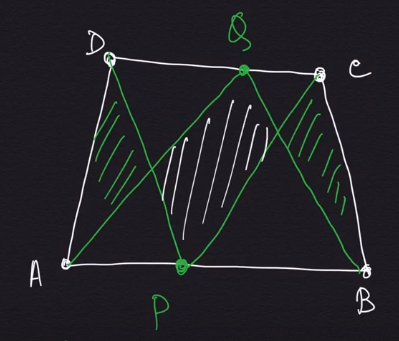How Cheenta works to ensure student success?
Explore the Back-StoryMahalanobis Olympiad is suitable for College and University Students, interested in Statistics and Mathematics. Check out the resources for the Mahalanobis Olympiad in this post.

## Curriculum

• High School Mathematics
• Calculus and Linear Algebra
• Probability
• Statistics

### High School Mathematics

• Coordinate Geometry
• Trigonometry
• Complex Numbers
• Permutation and Combinatorics

### Calculus and Linear Algebra

• Pre Calculus
• One Variable Calculus
• Matrices and Determinants

### Probability

• Classical Probability
• Conditional Probability
• Bayes Theorem
• Random Variables
• Joint Random Variables
• Conditional Expectation
• Simulation

### Statistics

• Parametric Estimation
• Hypothesis Testing
• Simple and Multiple Linear Regression
• Non Parametric Estimation
• Linear Models

## Reference Books

• Calculus and Analytical Geometry by Thomas Finney
• Linear Algebra and its Applications by Gilbert Strang
• Statistics by Freedman, Purves, Pisani
• Introduction to Probability by J.K. Blitzstein, Jessica Hwang
• Mathematical Statistics and Data Analysis by John A Rice
• NCERT Books (till Standard 12)
• Challenges and Thrills of Pre College Mathematics

Mahalanobis Olympiad is suitable for College and University Students, interested in Statistics and Mathematics. Check out the resources for the Mahalanobis Olympiad in this post.

## Curriculum

• High School Mathematics
• Calculus and Linear Algebra
• Probability
• Statistics

### High School Mathematics

• Coordinate Geometry
• Trigonometry
• Complex Numbers
• Permutation and Combinatorics

### Calculus and Linear Algebra

• Pre Calculus
• One Variable Calculus
• Matrices and Determinants

### Probability

• Classical Probability
• Conditional Probability
• Bayes Theorem
• Random Variables
• Joint Random Variables
• Conditional Expectation
• Simulation

### Statistics

• Parametric Estimation
• Hypothesis Testing
• Simple and Multiple Linear Regression
• Non Parametric Estimation
• Linear Models

## Reference Books

• Calculus and Analytical Geometry by Thomas Finney
• Linear Algebra and its Applications by Gilbert Strang
• Statistics by Freedman, Purves, Pisani
• Introduction to Probability by J.K. Blitzstein, Jessica Hwang
• Mathematical Statistics and Data Analysis by John A Rice
• NCERT Books (till Standard 12)
• Challenges and Thrills of Pre College Mathematics

This site uses Akismet to reduce spam. Learn how your comment data is processed.

### Knowledge Partner